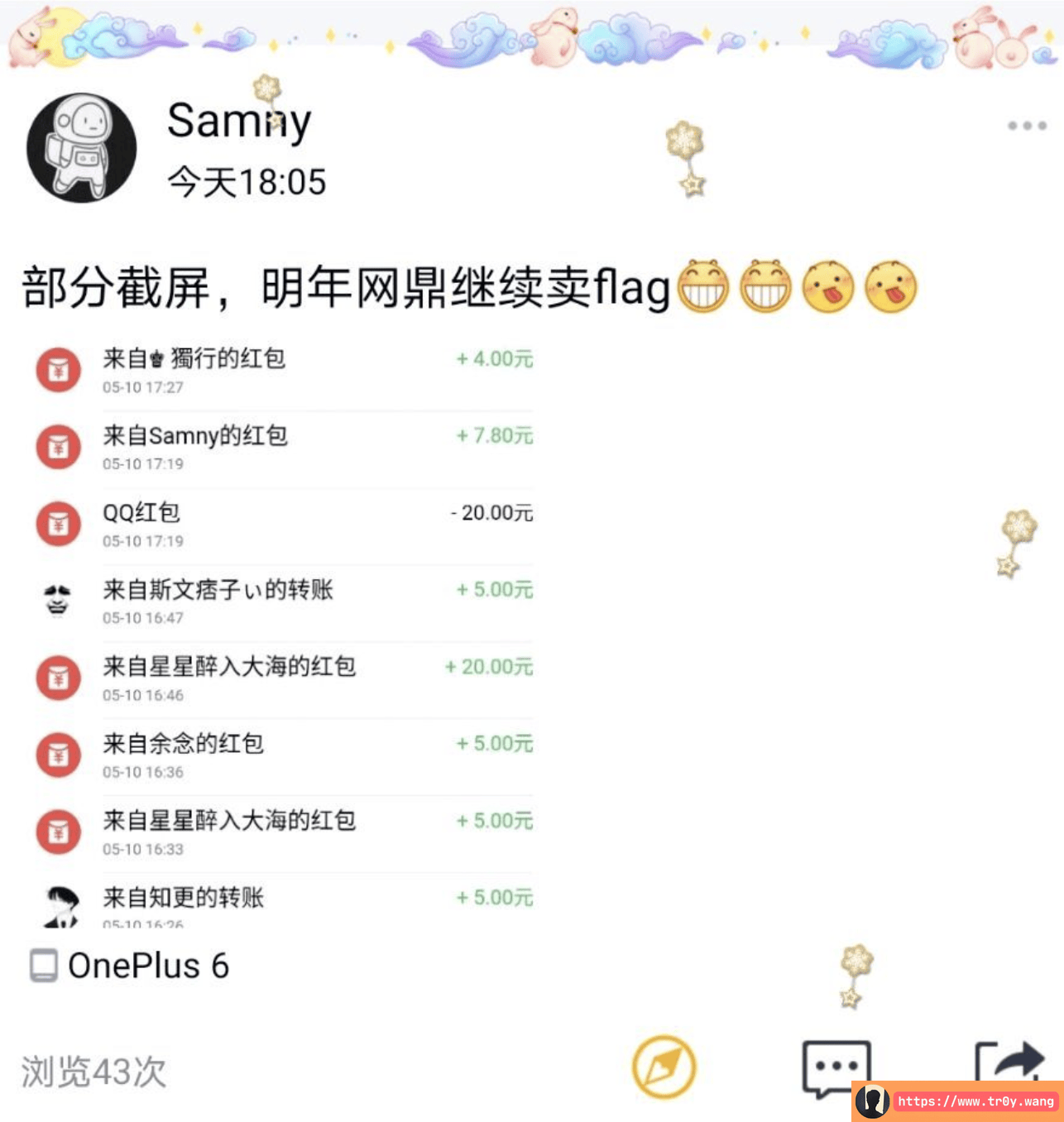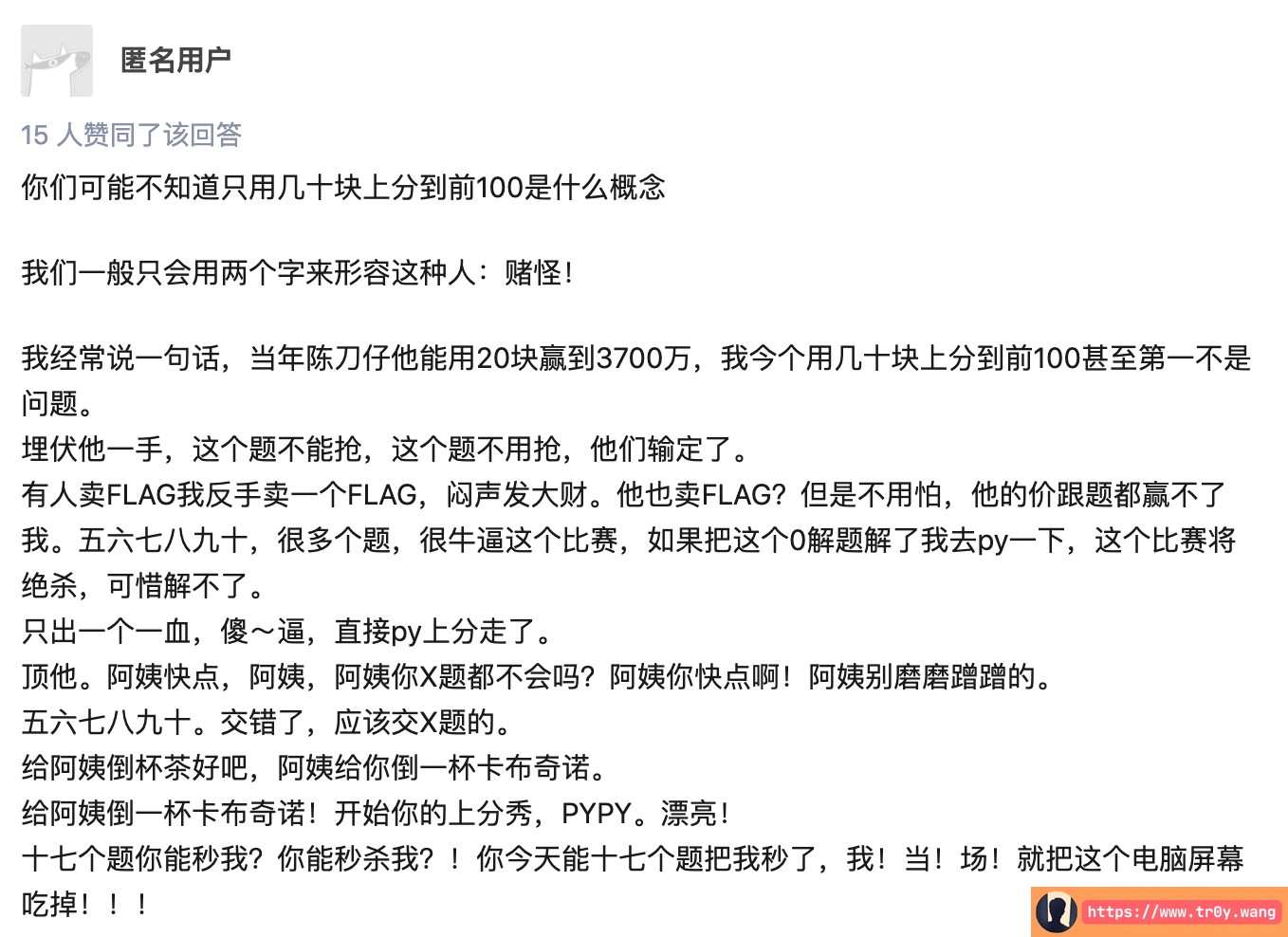## 过程

### open_box

...
<build-in md5>


### easy_wa

3723147309
8034322053911434180
49745872180417846725334070082004720258
52533909732625715277851750493149794582946123291447879643150522322430787956274


from secret import flag
from os import urandom as ua
import binascii
from libnum import s2n

# 原来是 lambda，改了一下，不影响
def hlen(x): return len(hex(x)[2:].strip('L'))

magic = 32805

def calc(m, p):
res = 0
lens = hlen(p)
for i in bin(m)[2:]:
res *= 2
res ^= m if i == '1' else 0
res ^= p if hlen(res) == lens else 0
return res

# pow_check()
# token_check()

assert len(flag) <= 64
if len(flag) != 64:
for i in range(64-len(flag)):
flag += ua(1)

r = 4
for i in range(4):
print(calc(s2n(flag[:r]), magic + (1 << r*8)))
flag = flag[r:]
r *= 2


flag: 3723147309
{6372edc: 8034322053911434180
74529ccec2ed74d7: 49745872180417846725334070082004720258


712af599a}|\xb6\x0f\x8f\xf4v\xbaC\xad\xb7\xb0^\xdf\xe13\x87\xf5\xe4\x8c\xb7\x0cf: 52533909732625715277851750493149794582946123291447879643150522322430787956274flag{6372edc74529ccec2ed74d7712af599a}


## 一些想法

py